# Iteration Methods

Consider the function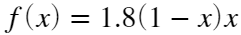and the iteration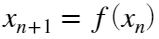started at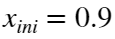initial value. Compute the first 10 iterates.
f = @(x) 1.8*(1-x).*x; %iteration function
x(1) = 0.9; %initial value
numIter=50;
for i = 1:numIter %number of iterates
x(i+1) = f(x(i));
end
%plot iterations points
X = x;
Y = zeros(1, length(x));
scatter(X,Y)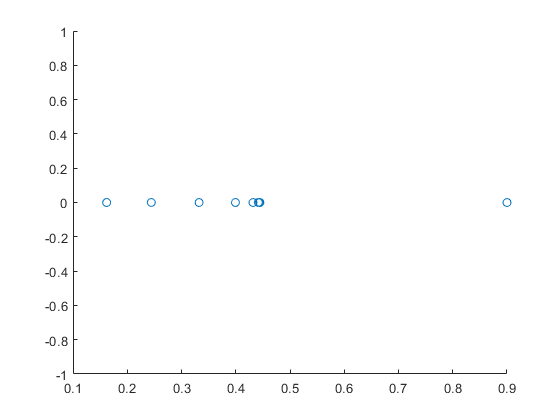% Hint: Try this other representation
% szPoints = linspace(300,1,length(x)); %size of the points
% colorPoints = linspace(1,10,length(x)); %colors for each point
% scatter(X,Y,szPoints,colorPoints)

## Exercise 0:

Compute the fixed point for the iterate function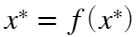and determine how many iterates are needed to achieve a precision of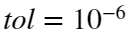when approximate numerically the fixed point, that is: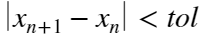.
Hint: You must use a while loop instead of a for loop.
Sol: n=13

## Exercise 1:

Compute the number of terms needed to approximate the sum of the Harmonic seriewith a precision of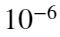Sol: n = 1.0e+6, suma = 1.644933066848770e+00

## Exercise 2:

Do the Fibonacci iteration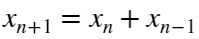starting from the initial values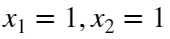. Compute the ratiobetween two consecutive values and verify that it converges to the Golden ratio, Gr. How many iterations you need to have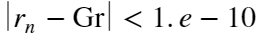?
Sol: n = 25

## Aitken acceleration

One possibility of accelerating the convergence of an iteration procedure is to use the Aitken method. The idea is to create a new succesion from the present iteration which converges faster:## Exercise 3:

Using the iteration function. Compute the succcesive values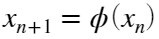and also the Aitken succession. Use x0=2, maxIter=100, tol= 1.e-10 .
Sol:
iter = 0, x0 = 2.0000000000000000e+00
iter = 1, xAitk = 1.0191337034629475e+00
iter = 2, xAitk = 1.0000257973776343e+00
iter = 3, xAitk = 1.0000000000481342e+00
iter = 4, xAitk = 9.9999999999999989e-01

# Graphical iteration: Logistic function (example of Chaos)

From a geometrical point of view a fixed point is the intersection of the line y = x with the plot of the funtion y = f(x).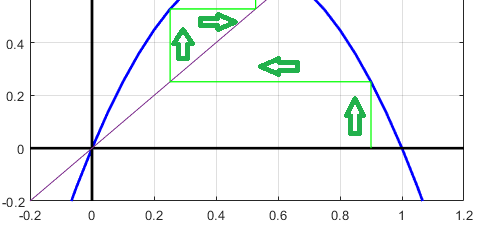Graphically the iteration procedure consist in two steps:
• Projection on the function: make a line from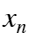to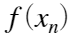• Change x-y values: make a line from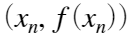to the diagonal line
L = 2.8;
logist = @(x,L) L.*(1-x).*x;
x = -0.2:0.05:1.2;
y = logist(x,L);
%----------------------------------
% Plot function and coordinate axis
%----------------------------------
figure()
% Plot iteration function
plot (x,y,'Color','b','LineWidth',2);
hold on;
axis([-0.2 1.2 -0.2 1.1])
grid
% Plot coordinate axis
plot([-0.2 1.2],[0 0],'Color','k','LineWidth',2)
plot([0 0],[-0.2 2],'Color','k','LineWidth',2)
% Plot Diagonal line
plot([-0.2 2],[-0.2, 2]);
%--------------------------------
% Start and plot iterates:
%--------------------------------
% Initial point
x0 = 0.9;
y0 = 0.0;
% Iteration loop
for i = 1:10
y1 = logist(x0,L);
plot([x0 x0],[y0 y1],'Color','g','LineWidth',1); %go to the function
hold on;
pause(0.3)
x1 = y1;
plot([x0 x1],[y1 y1],'Color','g','LineWidth',1); %go to the diagonal
pause(0.3)
% update iteration
y0 = y1;
x0 = x1;
drawnow;
end
text(0.85,0.85,['Param = ' num2str(L,'%.4f')])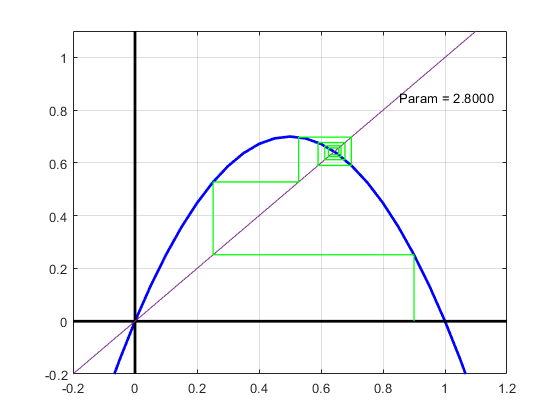## Exercise 4:

Plot the continuous evolution of the logistic iteration when going form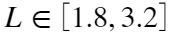(start a new plot for each L). Choose always the initial point x0= 0.9 and do 100 iterates for each L.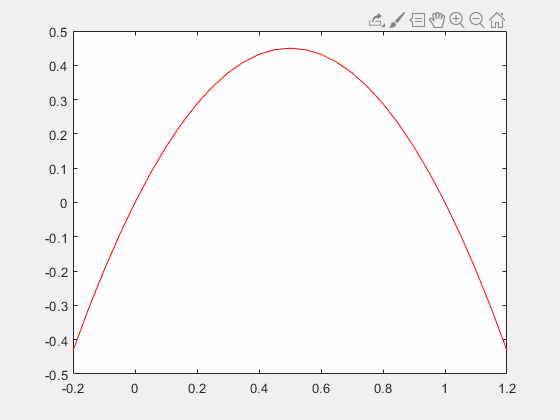## Exercise 5:

Plot the Biffurcation Diagram of Feigenbaum. It consists in plotting the logistic iterates (n=200) for different values of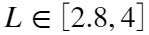. To avoid initial inestabilities we will take always the initial point x0= 0.9 but we will plot only the points from the 50th iterate to the 200th. The final plot is shown below.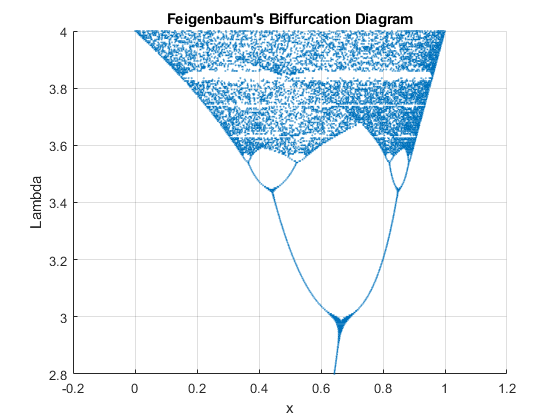(c) Numerical Factory - 2020